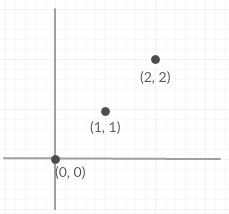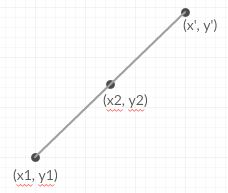# Reflection of a point at 180 degree rotation of another point

Given two points coordinates (x1, y1) and (x2, y2)on 2D plane. The task is to find the reflection of (x1, y1) at 180 degree rotation of (x2, y2).

Examples:

```Input : x1 = 0, y1 = 0, x2 = 1, y2 = 1
Output : (2, 2)Input : x1 = 1, y1 = 1, x2 = 2, y2 = 2
Output : (3, 3)
```

Let the reflection point of point (x1, y1) about (x2, y2) be (x’, y’).
For (x’, y’) be the 180 degree rotation of point (x1, y1) around point (x2, y2), they all must be collinear i.e all the three point must lie on a same straight line. Also, observe (x2, y2) will became mid point between (x1, y1) and (x’, y’).So,
x’ – x2 = x2 – x1
y’ – y2 = y2 – y1

x’ = 2 * x2 – x1
y’ = 2 * y2 – y1

Below is the implementation of this approach:

## C++

 `// CPP Program tof find the 180 degree reflection ` `// of one point around another point. ` `#include ` `using` `namespace` `std; ` ` `  `void` `findPoint(``int` `x1, ``int` `y1, ``int` `x2, ``int` `y2) ` `{ ` `    ``cout << ``"("` `<< 2 * x2 - x1 << ``", "`  `         ``<< 2 * y2 - y1 << ``")"``; ` `} ` ` `  ` `  `int` `main() ` `{ ` `    ``int` `x1 = 0, y1 = 0, x2 = 1, y2 = 1; ` `    ``findPoint(x1, y1, x2, y2); ` `    ``return` `0; ` `} `

## Java

 `// Java Program to find the 180 degree  ` `// reflection of one point around  ` `// another point. ` `class` `GFG { ` `     `  `    ``static` `void` `findPoint(``int` `x1, ``int` `y1, ` `                          ``int` `x2, ``int` `y2) ` `    ``{ ` `        ``System.out.println(``"("` `+ (``int``)(``2` `* x2 - x1) ` `               ``+ ``","` `+ (``int``)(``2` `* y2 - y1 ) + ``" )"``); ` `    ``} ` `     `  `    ``// Driver code ` `    ``public` `static` `void` `main(String args[]) ` `    ``{ ` `        ``int` `x1 = ``0``, y1 = ``0``, x2 = ``1``, y2 = ``1``; ` `         `  `        ``findPoint(x1, y1, x2, y2); ` `    ``} ` `} ` ` `  `// This code is contributed by Arnab Kundu. `

## Python3

 `# Python3 Program for find the 180 ` `# degree reflection of one point ` `# around another point. ` ` `  `def` `findPoint(x1, y1, x2, y2): ` `    ``print``(``"("` `, ``2` `*` `x2 ``-` `x1 , ``","``,  ` `                ``2` `*` `y2 ``-` `y1 ,``")"``); ` ` `  `# Driver Code ` `x1 ``=` `0``; ` `y1 ``=` `0``;  ` `x2 ``=` `1``;  ` `y2 ``=` `1``; ` `findPoint(x1, y1, x2, y2); ` ` `  `# This code is contributed by mits `

## C#

 `// C# Program to find the 180 degree reflection ` `// of one point around another point. ` `using` `System; ` ` `  `public` `class` `GFG { ` `     `  `    ``static` `void` `findPoint(``int` `x1, ``int` `y1,  ` `                          ``int` `x2, ``int` `y2) ` `    ``{ ` `        ``Console.WriteLine(``"("` `+ (``int``)(2 * x2 - x1)  ` `               ``+ ``","` `+ (``int``)(2 * y2 - y1 ) + ``" )"``); ` `    ``} ` `     `  `    ``// Driver code ` `    ``static` `public` `void` `Main(String []args) ` `    ``{ ` `        ``int` `x1 = 0, y1 = 0, x2 = 1, y2 = 1; ` `         `  `        ``findPoint(x1, y1, x2, y2); ` `    ``} ` `} ` ` `  `// This code is contributed by Arnab Kundu. `

## PHP

 ` `

Output:

```(2, 2)
```

Time Complexity : O(1)

Attention reader! Don’t stop learning now. Get hold of all the important DSA concepts with the DSA Self Paced Course at a student-friendly price and become industry ready.

My Personal Notes arrow_drop_upCheck out this Author's contributed articles.

If you like GeeksforGeeks and would like to contribute, you can also write an article using contribute.geeksforgeeks.org or mail your article to contribute@geeksforgeeks.org. See your article appearing on the GeeksforGeeks main page and help other Geeks.

Please Improve this article if you find anything incorrect by clicking on the "Improve Article" button below.

Improved By : andrew1234, vt_m, Mithun Kumar